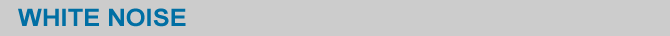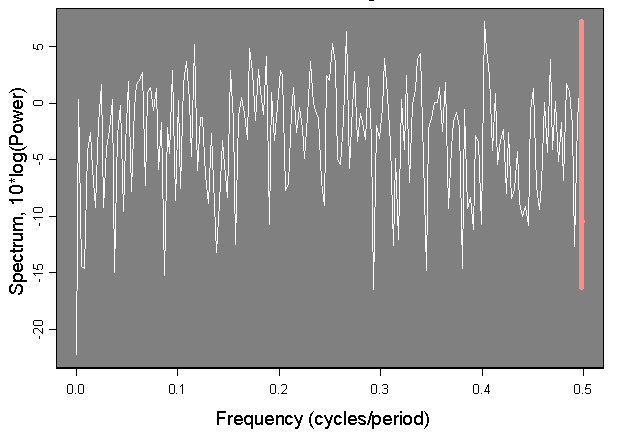A process that has a normal independent identical distirbution with mean zero and given variance is called white noise. White noise is characterized by a flat spectrum, i.e. the variance is approximately the same at all frequencies. An example is shown in Figure 1.Figure 1. Spectrum of white noise: sample of 365 members from a normal distribution with mean 0 and variance 1. Pink bar shows the spectrum analysis 95% confidence interval limits. No frequency contributes to a power that would exceed the confidence interval limits.